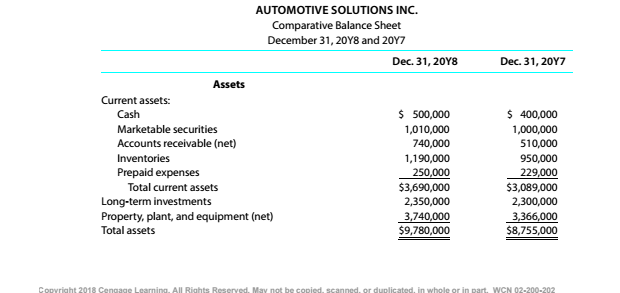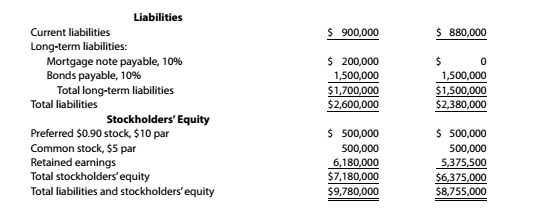Chapter 9, Problem 9.4.8P

Chapter
Section
Textbook Problem

Twenty metrics of liquidity, solvency, and profitabilityThe comparative financial statements of Automotive Solutions Inc. are as follows. The market price of Automotive Solutions Inc. common stock was $119.70 on December 31, 20Y8Instructions Debt ratio To determine Concept Introduction: Debt Ratio: Debt ratio is the relationship between the Total liabilities and Total assets of a corporation. Debt ratio shows the part of assets financed by debts. It is calculated by dividing total liabilities by total assets. The formula of debt ratio is as follows: Debt Ratio = Total LiabilitiesTotal Assets To Calculate: The Debt Ratio. Explanation The Debt Ratio is calculated as follows:  20Y8 20Y7 Total Liabilities (A)$ 2,600,000

Still sussing out bartleby?

Check out a sample textbook solution.

See a sample solution

The Solution to Your Study Problems

Bartleby provides explanations to thousands of textbook problems written by our experts, many with advanced degrees!

Get Started